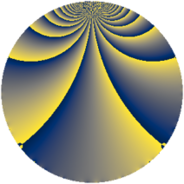# Properties

 Label 684.1.qLevel $684$ Weight $1$ Character orbit 684.q Rep. character $\chi_{684}(163,\cdot)$ Character field $\Q(\zeta_{6})$ Dimension $8$ Newform subspaces $1$ Sturm bound $120$ Trace bound $0$

# Related objects

## Defining parameters

 Level: $$N$$ $$=$$ $$684 = 2^{2} \cdot 3^{2} \cdot 19$$ Weight: $$k$$ $$=$$ $$1$$ Character orbit: $$[\chi]$$ $$=$$ 684.q (of order $$6$$ and degree $$2$$) Character conductor: $$\operatorname{cond}(\chi)$$ $$=$$ $$76$$ Character field: $$\Q(\zeta_{6})$$ Newform subspaces: $$1$$ Sturm bound: $$120$$ Trace bound: $$0$$

## Dimensions

The following table gives the dimensions of various subspaces of $$M_{1}(684, [\chi])$$.

Total New Old
Modular forms 24 12 12
Cusp forms 8 8 0
Eisenstein series 16 4 12

The following table gives the dimensions of subspaces with specified projective image type.

$$D_n$$ $$A_4$$ $$S_4$$ $$A_5$$
Dimension 0 0 8 0

## Trace form

 $$8q + O(q^{10})$$ $$8q + 4q^{10} + 4q^{13} + 4q^{16} + 4q^{22} - 4q^{25} + 4q^{28} - 8q^{37} - 4q^{40} - 8q^{46} - 4q^{61} - 4q^{70} - 4q^{73} - 4q^{76} - 8q^{88} + O(q^{100})$$

## Decomposition of $$S_{1}^{\mathrm{new}}(684, [\chi])$$ into newform subspaces

Label Dim. $$A$$ Field Image CM RM Traces $q$-expansion
$$a_2$$ $$a_3$$ $$a_5$$ $$a_7$$
684.1.q.a $$8$$ $$0.341$$ $$\Q(\zeta_{24})$$ $$S_{4}$$ None None $$0$$ $$0$$ $$0$$ $$0$$ $$q-\zeta_{24}^{5}q^{2}+\zeta_{24}^{10}q^{4}+(\zeta_{24}^{5}+\zeta_{24}^{11}+\cdots)q^{5}+\cdots$$